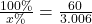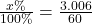## x% of 60 is 3.006 find it out for me and you better be smart

Question

x% of 60 is 3.006 find it out for me and you better be smart

in progress 0
3 months 2021-07-30T16:42:41+00:00 1 Answers 1 views 0

5.01

Step-by-step explanation:

rude..

3.006:60*100 =

(3.006*100):60 =

300.6:60 = 5.01

Now we have: 3.006 is what percent of 60 = 5.01

Question: 3.006 is what percent of 60?

Percentage solution with steps:

Step 1: We make the assumption that 60 is 100% since it is our output value.

Step 2: We next represent the value we seek with x.

Step 3: From step 1, it follows that 100%=60.

Step 4: In the same vein, x%=3.006.

Step 5: This gives us a pair of simple equations:

100%=60(1).

x%=3.006(2).

Step 6: By simply dividing equation 1 by equation 2 and taking note of the fact that both the LHS

(left hand side) of both equations have the same unit (%); we haveStep 7: Taking the inverse (or reciprocal) of both sides yields> x=5.01%

Therefore, 3.006 is 5.01% of 60.